## UGC NET CS 2017 Nov- paper-3

 Question 1
In 8085 microprocessor which of the following flag(s) is (are) affected by an arithmetic operation ?
 A AC flag Only B CY flag Only C Z flag Only D AC, CY, Z flags
Computer-Organization       Microprocessor
Question 1 Explanation:
AC Flag: This flag is set if thee is a carry to fifth bit from the fourth bits if two numbers.Question 2
In 8085 microprocessor the address bus is of __________ bits.
 A 4 B 8 C 16 D 32
Computer-Organization       Microprocessor
Question 2 Explanation:
In 8085 microprocessor the address bus is of 16 bits and data bus is 8 bits.
 Question 3
In the architecture of 8085 microprocessor match the following:A (a)-(iv), (b)-(i), (c)-(ii) B (a)-(iii), (b)-(iv), (c)-(ii) C (a)-(ii), (b)-(iii), (c)-(i) D (a)-(i), (b)-(ii), (c)-(iv)
Computer-Organization       Microprocessor
Question 3 Explanation:
Processing unit→ ALU
Arithmetic logic unit(ALU) is a fundamental building block of many types of computing circuits, including the central processing unit (CPU) of computers, FPUs, and graphics processing units (GPUs).
Instruction unit →Timing and control
Timing and Control unit is responsible for hardwired control unit and Microprogrammed control unit
Storage and Interface unit → General purpose Register
The general purpose register can store a data (or) a memory location address.
 Question 4
Which of the following addressing mode is best suited to access elements of an array of contiguous memory locations ?
Data-Structures       Arrays
Question 4 Explanation:
Index mode:
The address of the operand is obtained by adding to the contents of the general register (called index register) a constant value. The number of the index register and the constant value are included in the instruction code.
→ Index Mode is used to access an array whose elements are in successive memory locations (or) contiguous memory locations.
 Question 5
Which of the following is correct statement ?
 A In memory - mapped I/O, the CPU can manipulate I/O data residing in interface registers that are not used to manipulate memory words. B The isolated I/O method isolates memory and I/O addresses so that memory address range is not affected by interface address assignment. C In asynchronous serial transfer of data the two units share a common clock. D In synchronous serial transmission of data the two units have different clocks.
Computer-Organization       Isolated-vs-Memory-mapped-I/O
Question 5 Explanation:
FALSE: In memory - mapped I/O, the CPU can manipulate I/O data residing in interface registers that are used to manipulate memory words.
TRUE: The isolated I/O method isolates memory and I/O addresses so that memory address range is not affected by interface address assignment.
FALSE: In asynchronous serial transfer of data the two units share a different clock.
FALSE: In synchronous serial transmission of data the two units have common clocks.
 Question 6
A microinstruction format has micro-ops field which is divided into three subfields F1, F2, F3 each having seven distinct micro-operations, condition field CD for four status bits, branch field BR having four options used in conjunction with address field ADF. The address space is of 128 memory locations. The size of micro-instruction is:
 A 17 B 20 C 24 D 32
Computer-Organization       Microprogrammed-Control-Unit
Question 6 Explanation:
Given data, -- Micro-ops field F1 = 7 distinct micro operations
-- Micro-ops field F2 = 7 distinct micro operations
-- Micro-ops field F3 = 7 distinct micro operations
-- Condition field(CD) = 4 status bits
-- Branch Field(BR) = 4 options
-- Address space =128 memory locations
-- Size of micro operation=?
Given microinstruction formatQuestion 7
Consider the following four schedules due to three transactions (indicated by the subscript) using read and write on a data item X, denoted by r(X) and w(X) respectively. Which one of them is conflict serializable ?
S1: r1(X); r2(X); w1(X); r3(X); w2(X)
S2: r2(X); r1(X); w2(X); r3(X); w1(X)
S3: r3(X); r2(X); r1(X); w2(X); w1(X)
S4: r2(X); w2(X); r3(X); r1(X); w1(X)
 A S1 B S2 C S3 D S4
Database-Management-System       Transactions
Question 7 Explanation: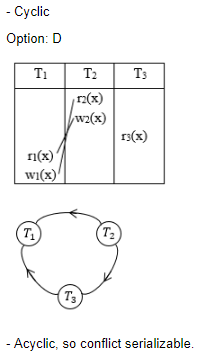Question 8
Suppose a database schedule S involves transactions T1, T2, .............,Tn. Consider the precedence graph of S with vertices representing the transactions and edges representing the conflicts. If S is serializable, which one of the following orderings of the vertices of the precedence graph is guaranteed to yield a serial schedule ?
 A Topological order B Depth - first order C Breadth - first order D Ascending order of transaction indices
Database-Management-System       Transactions
Question 8 Explanation:
If a schedule is conflict serializable then no cycle in precedence graph should be present.
But BFS and DFS are also possible for cyclic graphs.
And topological sort is not possible for cyclic graph.
Moreover option (D) is also wrong because in a transaction with more indices might come before lower one.
 Question 9
If every non-key attribute is functionally dependent on the primary key, then the relation is in __________ .
 A First normal form B Second normal form C Third normal form D Fourth normal form
Database-Management-System       Normalization
Question 9 Explanation:
1NF : A relation is in 1NF if it does not contain multi-value attributes and composite attributes.
2NF: A relation is in 2NF is there is no partial dependency exist in the relation. i.e.
primary_key → non-key attribute
prime_attribute → non-key attribute.
3NF : A relation is in 3NF if there is no transitive dependency or we can say that a relation is in 3NF if either LHS of a functional dependency is a super key or the RHS of the functional dependency is a prime key attribute.
4NF : A relation is in 4NF if there is no multivalued dependency exist in the relation.
Hence the correct answer is option (C)
NOTE: A prime key attribute is a attribute which is the part of primary key attributes. For example: If ABC is the the primary key then A,B,C, AB, AC, BC are the prime key attributes.
 Question 10
Consider a relation R (A, B, C, D, E, F, G, H), where each attribute is atomic, and following functional dependencies exist.
CH → G
A → BC
B → CFH
E → A
F → EG
The relation R is __________ .
 A in 1NF but not in 2NF B in 2NF but not in 3NF C in 3NF but not in BCNF D in BCNF
Database-Management-System       Normalization
Question 10 Explanation:
The attribute D is not part of any FD's. So, D can be a candidate key or it may be part of the candidate key.
Now D+ = {D}.
Hence we have to add A,B,C,E,F,G,H to D and check which of them are Candidate keys of size 2.
BD+ = {ABCDEFGH}
ED+ = {ABCDEFGH}
FD+= {ABCDEFGH}
But CD+, GD+ and HD+ does not give all the attributes hence CD, GD and HD are not candidate keys.
Here Candidate keys are AD, BD, ED and FD.
A → BC, B → CFH and F → EG etc are partial dependencies.
So given relation is in 1NF, but not in 2NF.
 Question 11
Given two relations R1(A, B) and R2(C, D), the result of following query Select distinct A, B from R1, R2 is guaranteed to be same as R1 provided one of the following condition is satisfied.
 A R1 has no duplicates and R2 is empty. B R1 has no duplicates and R2 is non - empty. C Both R1 and R2 have no duplicates. D R2 has no duplicates and R1 is non - empty.
Database-Management-System       SQL
Question 11 Explanation:
Option(A): Cartesian product with a empty table will result in zero tuple because we can't have any ordered pair with a empty table. Hence option(A) is incorrect option.
Option(B):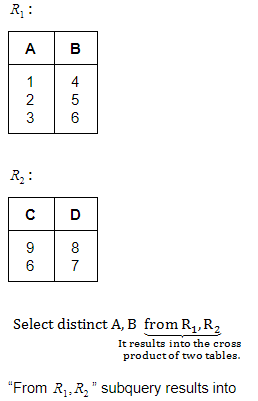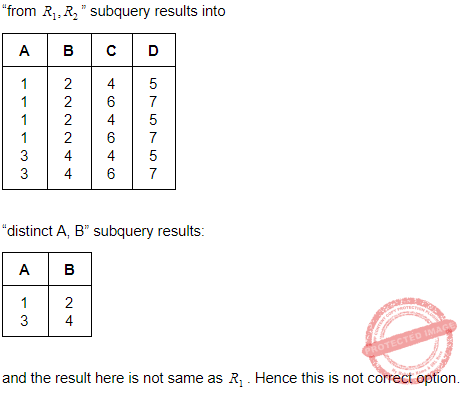Question 12
Consider a schema R(A, B, C, D) and following functional dependencies.
A → B
B → C
C → D
D → B
Then decomposition of R into R1(A, B), R2(B, C) and R3(B, D) is __________ .
 A Dependency preserving and lossless join. B Lossless join but not dependency preserving. C Dependency preserving but not lossless join. D Not dependency preserving and not lossless join.
Database-Management-System       Functional-Dependency
Question 12 Explanation:
(A, B) (B, C) - common attribute is (B) and due to B→C, B is a key for (B, C) and hence ABC can be losslessly decomposed into (A, B)and (B, C).
(A, B, C) (B, D) - common attributes is B and B→D is a FD (via B→C, C→D), and hence, B is a key for (B, D). So, decomposition of (A, B, C, D) into (A, B, C) (B, D) is lossless.
Thus the given decomposition is lossless.
The given decomposition is also dependency preserving as the dependencies A→B is present in (A, B), B→C is present in (B, C), D→B is present in (B, D) and C→D is indirectly present via C→B in (B, C) and B→D in (B, D).
 Question 13
Which of the following is not a component of Memory tube display ?
 A Flooding gun B Collector C Ground D Liquid Crystal
Computer-Graphics       Display-System
Question 13 Explanation:
Memory tube display components:
1. Connector Pins
2. Electron Gun
3. Base
4. Focusing System
5. Control Grid Voltage
6. X/Y Deflect
7. Phosphor
8. Collector
9. Ground
Note: Liquid crystal is not a component of memory tube display
.
 Question 14
Which of the following is not true in case of Oblique Projections?
 A Parallel projection rays are not perpendicular to the viewing plane. B Parallel lines in space appear parallel on the final projected image. C Used exclusively for pictorial purposes rather than formal working drawings. D Projectors are always perpendicular to the plane of projection.
Computer-Graphics       Oblique-Projections
 Question 15
With respect to CRT, the horizontal retrace is defined as:
 A The path an electron beam takes when returning to the left side of the CRT. B The path an electron beam takes when returning to the right side of the CRT. C The technique of turning the electron beam off while retracing. D The technique of turning the electron beam on/off while retracing.
Computer-Graphics       Display-System
 Question 16
Find the equation of the circle x2+y2=1 in terms of x'y' coordinates, assuming that the xy coordinate system results from a scaling of 3 units in the x' direction and 4 units in the y' direction.
 A 3(x')2 + 4(y')2 = 1 B (x'/3)2 + (y'/4)2 = 1 C (3x')2 + 4(y')2 = 1 D 1/3(x')2 + 1/4(y')2 = 1
Engineering-Mathematics       Co-ordinate-Geometry
 Question 17
Find the normalization transformation that maps a window whose lower left corner is at (1, 1) and upper right corner is at (3, 5) onto a viewport that is the entire normalized device screen.
 AB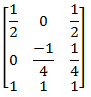CD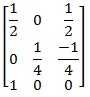Database-Management-System       Normalization
 Question 18
The three aspects of Quantization, programmers generally concerned with are:
 A Coding error, Sampling rate and Amplification B Sampling rate, Coding error and Conditioning C Sampling rate, Aperture time and Coding error D Aperture time, Coding error and Strobing
Computer-Networks       Quantization
There are 18 questions to complete.

Register Now